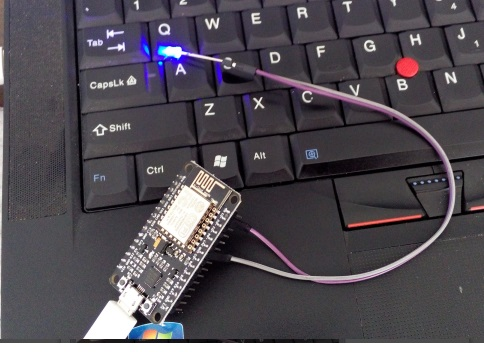# Subsection 4.3: PWM Function

The file name is "pwm.lua".

In NodeMCU, D1~12 pins have the PWM function (excluding D0). PWM frequency can cover 1~1000Hz, the cycle scope is 0~1023(i.e., 0%~100%).

In "init.lua", the "fileName.lua" is revised as "pwm.lua", and "fileName.lc" is revised as "pwm.lc". After revision, and then download it. The code for PWM is as follows.

``````1     print("PWM Function test")
2     pwm.setup(1,1000,1023);
3     pwm.start(1);
4
5     local r=512;
6     local flag=1;
7     tmr.alarm(2,100,1,function()
8         pwm.setduty(1,r);
9         if flag==1 then
10         r=r-50;        if r《0 then flag=0 r=0 end
11         else
12         r= r+50;    if r>1023 then flag=1 r=1023 end
13     end
14     end)
``````

line 1: print character;

line 2: set the 1st port as 1000Hz, the cycle is 1023;

line 3: start the PWM output;

line 5-14: set timer2, add or reduce cycle "r" after each 100ms; The variable flag can remember the change.

Tips: when download, can see the change of the ouput volage from port D1 by using multimeter and/or oscilloscope. Another way, if a LED is inserted between D1 and GND. It can see the change of brightness.Tips：for LED, the longer pin is positive and connected to D1; and the short pin is negative, and connect to GND. The log is as follows.

``````1     NodeMCU 0.9.6 build 20150406  powered by Lua 5.1.4
2
3
4     ESP8266 Started
5     Compile File:pwm.lua
6     Remove File:pwm.lua
7     PWM Function test
8     >
``````